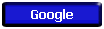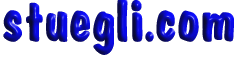# Newton's First Law##### "an object will remain at rest or in uniform motion in a straight line unless acted upon by an external force."

It may be seen as a statement about inertia, that objects will remain in their state of motion unless a force acts to change the motion. Any change in motion involves an acceleration, and then Newton's Second Law applies; in fact, the First Law is just a special case of the Second Law for which the net external force is zero.

Newton's First Law contains implications about the fundamental symmetry of the universe in that a state of motion in a straight line must be just as "natural" as being at rest. If an object is at rest in one frame of reference, it will appear to be moving in a straight line to an observer in a reference frame which is moving by the object. There is no way to say which reference frame is "special", so all constant velocity reference frames must be equivalent.Copyright © 2005 -  S. B. EglI